International
Tables for
Crystallography
Volume B
Reciprocal space
Edited by U. Shmueli

International Tables for Crystallography (2010). Vol. B, ch. 1.3, pp. 43-44   | 1 | 2 |

## Section 1.3.2.6.5. The case of nonstandard period lattices

G. Bricognea

aGlobal Phasing Ltd, Sheraton House, Suites 14–16, Castle Park, Cambridge CB3 0AX, England, and LURE, Bâtiment 209D, Université Paris-Sud, 91405 Orsay, France

#### 1.3.2.6.5. The case of nonstandard period lattices

| top | pdf |

Let Λ denote the nonstandard lattice consisting of all vectors of the form, where theare rational integers andare n linearly independent vectors in. Let R be the corresponding lattice distribution:.

Let A be the nonsingularmatrix whose successive columns are the coordinates of vectorsin the standard basis of; A will be called the period matrix of Λ, and the mapping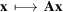will be denoted by A. According to Section 1.3.2.3.9.5we have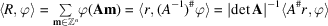for any, and hence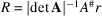. By Fourier transformation, according to Section 1.3.2.5.5,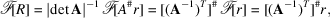which we write: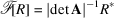withis a lattice distribution:associated with the reciprocal latticewhose basis vectorsare the columns of. Since the latter matrix is equal to the adjoint matrix (i.e. the matrix of co-factors) of A divided by det A, the components of the reciprocal basis vectors can be written down explicitly (see Section 1.3.4.2.1.1for the crystallographic case).

A distribution T will be called Λ-periodic iffor all; as previously, T may be writtenfor some motif distributionwith compact support. By Fourier transformation,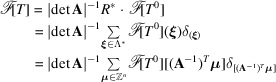so thatis a weighted reciprocal-lattice distribution, the weight attached to nodebeing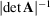times the valueof the Fourier transform of the motif.

This result may be further simplified if T and its motifare referred to the standard period latticeby defining t andso that,,. Then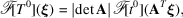hence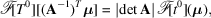so thatin nonstandard coordinates, whilein standard coordinates.

The reciprocity theorem may then be written: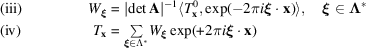in nonstandard coordinates, or equivalently:in standard coordinates. It gives an n-dimensional Fourier series representation for any periodic distribution over. The convergence of such series inwill be examined in Section 1.3.2.6.10.